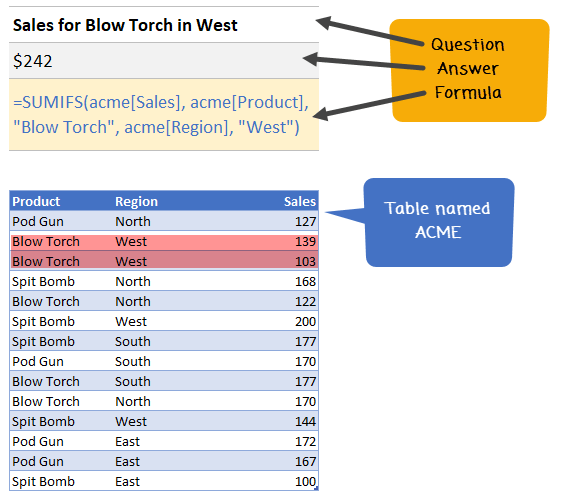How to write Excel SUMIFS Function? - KING OF EXCEL

## Saturday, December 14, 2019

Excel SUMIFS function is used to calculate the sum of values that meet any criteria. For example, you can calculate the total sales in east zone for product Pod Gun using SUMIFS formula.
• What is SUMIFS function and how to use it?
• Syntax for SUMIFS
• Using SUMIFS() with tables and structural references
• SUMIFS examples – simple, wild card
• Using SUMIFS() with date & time values
• Free sample file for SUMIFS formula
• More formulas for data analysis

### How to write Excel SUMIFS Function?

Using SUMIFS you can find the sum of values in your data that meet multiple conditions.
So, to get the sum of all the Blow Torches sold in North, we just write,
`=SUMIFS(D3:D16, B3:B16,"Blow Torch",C3:C16,"North")`
Similarly to find the podgun sales in East, just write,### SUMIFS function – Syntax and explanation:

SUMIFS formula takes a range for summing the values and at least one criteria range and criteria. You can specify as many as 127 conditions for summing your data.Imagine asking “how many spit bombs Hansolo sold in North region of Planet Naboo between long long ago and long ago that resulted in more than 25% profits?” and getting an instant answer.
The beauty of SUMIFS formula is that it works with wildcards too, just like its siblings – SUMIF and COUNTIF. So you can write formulas like,
`=SUMIFS(D3:D16,B3:B16,"Spit Bomb",C3:C16,"*th")` to get sum of spit bombs sold in North and South.

### Using SUMIFS() with tables

You can write SUMIFS function on either a range of data or on a table. When using with tables, you can simply apply structural references – ie TableName[Column Name] notation to specify the criteria columns. See this example:### SUMIFS Examples

Let’s say you have a table named ACME as pictured above. See these examples to understand how the function works.
• Sales for Blow Torch in West
• `=SUMIFS(acme[Sales], acme[Product], "Blow Torch", acme[Region], "West")`
• Total Sales above 150 in East
• `=SUMIFS(acme[Sales], acme[Sales],">150",acme[Region],"East")`
• Sales of North for all excluding Pod Gun
• `=SUMIFS(acme[Sales], acme[Region],"North",acme[Product],"<>Pod Gun")`
• `=SUMIFS(acme[Sales], acme[Product], "*B*")`

### Using SUMIFS() with Date & time values

When you have a column of dates, you can apply special operators like >, <, =, <> to specify a date range.
For example, to count total sales between March 2018 and May 2018, we can use
`=SUMIFS(acme[Sales], acme[Sales Date],">=1-Mar-2018", acme[Sales Date], "<=31-May-2018")`
You can either type the date in the formula or bring it from a cell. If you have two cells containing start and end date for your window of dates, you can use this formula.
`=SUMIFS(acme[Sales], acme[Sales Date],">=" & start_date_cell, acme[Sales Date], "<=" & end_date_cell)`
Replace start_date_cell and end_date_cell with actual cell references or names.

### Bonus:

Just like SUMIFS, there is COUNTIFS and AVERAGEIFS too in Excel. Once you know SUMIFS(), you can use all these other functions with ease.